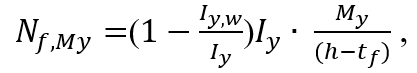# Force distribution in bolted cover plate splices, part 2

This is a continuation of the previous article about force distribution in bolted cover plate splices. We are going to further clarify how to derive the exact values of some forces and how to transfer them into the cover plates.

Load transfer for a vertical shear load, a horizontal shear load and a minor bending moment is straightforward and there is no need to comment on these. However, some clarification is required for other loads.

Let us start with a torsional moment. In the previous part, the torsional moment was replaced by two parallel forces HMx. The value of that force is equal to: HMx = Mx/(h-tf), where:

Mx - is the torsional moment; h - height of the beam; tf - beam flange thickness. The concept of this is known as a force couple.

Major bending moment can be distributed between the flanges and the web with their respective contributions to the overall moment of inertia and the force in each flange and the bending moment in the web can be calculated from the formulas below:Nf,My - force in each flange due to major bending moment,

Mw - moment occurring in the web,

My - design bending moment,

Iy - second moment of area about the major axis of the beam,

Iy,w - second moment of area of the web,

h - height of the beam,

tf - beam flange thickness.

It is a very common approach to neglect the bending moment within the web and just distribute all moment to the flanges. Although this is usually acceptable, it is safe to exactly distribute the moment, both to the flanges and to the web. One important example to consider are plate girders with slender webs, where the web resists a high shear load. It is essential to include a portion of the bending moment occurring in the web in order not to overdesign the element being considered.

Distribution of the axial force is quite simple and depends on the proportion of flange and web area to the area of the whole section and thus:Nf - force in each flange due to axial force,

Nw - force in the web due to axial force,

N - design axial force,

Aw - area of the web,

A - area of the section.

Having obtained the values of all forces and determined their distribution, we can now proceed to the next step and apply them to the two different elements: flange plates and web plates. Figure 1 shows a plan view of the splice with the relevant forces being applied to each flange.Figure 1.

Forces from horizontal shear load and torsional moment should be applied at the centreline of the splice. These act at an eccentricity – e – in relation to the centroid of bolts on one side of the splice. Other forces are applied at point 0.

Figure 2 shows the case for all the forces associated with the web zone design.Figure 2.

Vertical shear force is applied at the centreline of the splice and other forces at point 0. There are two very important issues to be considered:

Firstly, forces from the figures above acting at an eccentricity, shown in yellow, will cause additional moments in the flange or in the web at the centroid of the bolt groups. These moments will have to be added to the already occurring moments at point 0. To illustrate this, in Figure 2, we can move force V to point 0, but we need to add a moment equal to V*e to the already occurring moment Mw.

The second issue is that, we always consider only one side of the splice. It is a very common mistake to assume that the forces are distributed to all bolts on the two halves forming the splice. We take the full force occurring at the centreline of the splice and then we need to consider only one half of the splice. For example, in Figure 1 there is an axial force in the flange equal to Nf+Nf,My. To find a component acting on a single bolt, in our example we need to divide this force by 6, and not by 12.

Having all forces applied at point 0, we can now calculate the exact resultant values of shear forces for each bolt. This process has already been described in one of our previous articles about shear force distribution in bolts.

Tags: